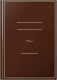# Non-Convex Programming for PolynomialsInstitute of Statistics, Texas A & M University, 1971 - 106 من الصفحات
The report develops a numerical solution to the problem of maximizing a polynomial, not necessarily concave, over the closure of a bounded domain in the n-dimensional Euclidean space. As an essential part of this solution algorithms for computing integrals of powers of polynomials are developed. For the general problem of maximizing a function over a region defined by the intersection of non-linear inequalities, a theorem which gives the point of maximum as well as the maximum value of the objective function is stated and proved. Again no assumption on the concavity of the non-linear functions is made. (Author).

### ما يقوله الناس -كتابة مراجعة

لم نعثر على أي مراجعات في الأماكن المعتادة.